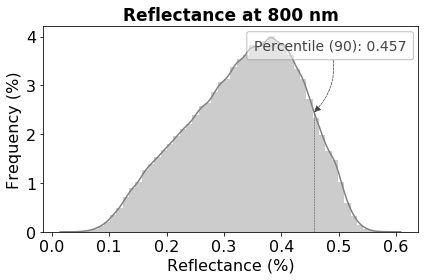# 8.1.4. hstools¶

class `hs_process.``hstools`(spyfile)[source]

Bases: `object`

Basic tools for manipulating Spyfiles and accessing their metadata.

Parameters

spyfile (`SpyFile` object) – The datacube being accessed and/or manipulated.

Methods Summary

 `clean_md_sets`([metadata]) Converts metadata items that are expressed as a list to be expressed as a dictionary. `del_meta_item`(metadata, key) Deletes metadata item from SpyFile object. `get_UTM`(pix_e_ul, pix_n_ul, utm_x, utm_y, …) Calculates the new UTM coordinate of cropped plot to modify the “map info” tag of the .hdr file. `get_band`(target_wl[, spyfile]) Finds the band number of the closest target wavelength. `get_band_index`(band_num) Subtracts 1 from `band_num` and returns the band index(es). `get_band_num`(band_idx) Adds 1 to `band_idx` and returns the band number(s). `get_band_range`(range_wl[, index, spyfile]) Retrieves the band index or band number for all bands within a wavelength range. `get_center_wl`(wl_list[, spyfile, wls]) Gets band numbers and mean wavelength from all wavelengths (or bands) in `wl_list`. `get_meta_set`(meta_set[, idx]) Reads metadata “set” (i.e., string representation of a Python set; common in .hdr files), taking care to remove leading and trailing spaces. `get_spectral_mean`(band_list[, spyfile]) Gets the spectral mean of a datacube from a list of bands. `get_wavelength`(target_band[, spyfile]) Returns actual wavelength of the closest target band. `load_spyfile`(spyfile) Loads a `SpyFile` (Spectral Python object) for data access and/or manipulation by the `hstools` class. `mask_array`(array, metadata[, thresh, …]) Creates a masked numpy array based on a threshold value. `mean_datacube`(spyfile[, mask]) Calculates the mean spectra for a datcube; if `mask` is passed (as a `modify_meta_set`(meta_set, idx, value) Modifies metadata “set” (i.e., string representation of a Python set; common in .hdr files) by converting string to list, then adjusts the value of an item by its index. `plot_histogram`(array[, fname_fig, title, …]) Plots a histogram with the percentile value labeled.

Methods Documentation

`clean_md_sets`(metadata=None)[source]

Converts metadata items that are expressed as a list to be expressed as a dictionary.

Parameters

metadata (`dict`, optional) – Metadata dictionary to clean

Returns

metadata_out (`dict`) – Cleaned metadata dictionary.

Return type

`dict`

Example

Load and initialize `hsio`

```>>> from hs_process import hsio
>>> fname_in = r'F:\nigo0024\Documents\hs_process_demo\Wells_rep2_20180628_16h56m_pika_gige_7-Convert Radiance Cube to Reflectance from Measured Reference Spectrum.bip.hdr'
>>> io = hsio(fname_in)
```

Create sample metadata with “wavelength” expressed as a list of strings

```>>> metadata = {'samples': 1300,
'lines': 617,
'bands': 4,
'file type': 'ENVI Standard',
'wavelength': ['394.6', '396.6528', '398.7056',
'400.7584']}
```

Clean metadata using `hstools.clean_md_sets`. Notice how wavelength is now expressed as a `str` representation of a `dict`, which is required for properly writing the metadata to the .hdr file via save_image() in Spectral Python.

```>>> io.tools.clean_md_sets(metadata=metadata)
{'samples': 1300,
'lines': 617,
'bands': 4,
'file type': 'ENVI Standard',
'wavelength': '{394.6, 396.6528, 398.7056, 400.7584}'}
```
`del_meta_item`(metadata, key)[source]

Deletes metadata item from SpyFile object.

Parameters
• metadata (`dict`) – dictionary of the metadata

• key (`str`) – dictionary key to delete

Returns

metadata (`dict`) – Dictionary containing the modified metadata.

Return type

`dict`

Example

Load and initialize `hsio`

```>>> from hs_process import hsio
>>> fname_in = r'F:\nigo0024\Documents\hs_process_demo\Wells_rep2_20180628_16h56m_pika_gige_7-Convert Radiance Cube to Reflectance from Measured Reference Spectrum.bip.hdr'
>>> io = hsio(fname_in)
```

```>>> metadata = {'samples': 1300,
'lines': 617,
'bands': 4,
'file type': 'ENVI Standard',
'map info': '{UTM, 1.0, 1.0, 421356.76707299997, 4844936.7317699995, 0.04, 0.04, 15, T, WGS-84, units  meters, rotation  0.000}',
'wavelength': ['394.6', '396.6528', '398.7056',
'400.7584']}
```

Delete “map info” from `metadata` using `hstools.del_met_item`

```>>> io.tools.del_meta_item(metadata, 'map info')
{'samples': 1827,
'lines': 617,
'bands': 4,
'file type': 'ENVI Standard',
'wavelength': ['394.6', '396.6528', '398.7056', '400.7584']}
```
`get_UTM`(pix_e_ul, pix_n_ul, utm_x, utm_y, size_x, size_y)[source]

Calculates the new UTM coordinate of cropped plot to modify the “map info” tag of the .hdr file.

Parameters
• pix_e_ul (`int`) – upper left column (easting) where image cropping begins.

• pix_n_ul (`int`) – upper left row (northing) where image cropping begins.

• utm_x (`float`) – UTM easting coordinates (meters) of the original image (from the upper left).

• utm_y (`float`) – UTM northing coordinates (meters) of the original image (from the upper left).

• size_x (`float`) – Ground resolved distance of the image pixels in the x (easting) direction (meters).

• size_y (`float`) – Ground resolved distance of the image pixels in the y (northing) direction (meters).

Returns

2-element `tuple` containing

• utm_x_new (`float`): The modified UTM x coordinate (easting) of cropped plot.

• utm_y_new (`float`): The modified UTM y coordinate (northing) of cropped plot.

Example

Load and initialize `hsio`

```>>> from hs_process import hsio
>>> fname_in = r'F:\nigo0024\Documents\hs_process_demo\Wells_rep2_20180628_16h56m_pika_gige_7-Convert Radiance Cube to Reflectance from Measured Reference Spectrum.bip.hdr'
>>> io = hsio(fname_in)
```

Retrieve UTM coordinates and pixel sizes from the metadata

```>>> map_info_set = io.spyfile.metadata['map info']
>>> utm_x = io.tools.get_meta_set(map_info_set, 3)
>>> utm_y = io.tools.get_meta_set(map_info_set, 4)
>>> spy_ps_e = float(map_info_set)
>>> spy_ps_n = float(map_info_set)
```

Calculate the UTM coordinates at the 100th easting pixel and 50th northing pixel using `hstools.get_UTM`

```>>> ul_x_utm, ul_y_utm = io.tools.get_UTM(100, 50,
utm_x, utm_y,
spy_ps_e,
spy_ps_n)
>>> ul_x_utm
441360.80707299995
>>> ul_y_utm
4855934.691769999
```
`get_band`(target_wl, spyfile=None)[source]

Finds the band number of the closest target wavelength.

Parameters
• target_wl (`int` or `float`) – the target wavelength to retrieve the band number for (required).

• spyfile (`SpyFile` object, optional) – The datacube being accessed and/or manipulated; if `None`, uses `hstools.spyfile` (default: `None`).

Returns

key_band (`int`) – band number of the closest target wavelength (`target_wl`).

Return type

`int`

Example

Load and initialize `hsio`

```>>> from hs_process import hsio
>>> fname_in = r'F:\nigo0024\Documents\hs_process_demo\Wells_rep2_20180628_16h56m_pika_gige_7-Convert Radiance Cube to Reflectance from Measured Reference Spectrum.bip.hdr'
>>> io = hsio(fname_in)
```

Use `hstools.get_band` to find the band number corresponding to 703 nm

```>>> io.tools.get_band(703, io.spyfile)
151
```
`get_band_index`(band_num)[source]

Subtracts 1 from `band_num` and returns the band index(es).

Parameters
• band_num (`int` or `list`) – the target band number(s) to retrieve

• band index for (the) –

Returns

band_idx (`int`) – band index of the passed band number (`band_num`).

Return type

`int` or `list`

Example

Load and initialize `hsio`

```>>> from hs_process import hsio
>>> fname_in = r'F:\nigo0024\Documents\hs_process_demo\Wells_rep2_20180628_16h56m_pika_gige_7-Convert Radiance Cube to Reflectance from Measured Reference Spectrum.bip.hdr'
>>> io = hsio(fname_in)
```

Using `hstools.get_band_index`, find the band index of the 4th, 43rd, and 111th bands

```>>> io.tools.get_band_index([4, 43, 111])
[3, 42, 110]
```
`get_band_num`(band_idx)[source]

Adds 1 to `band_idx` and returns the band number(s).

Parameters

band_idx (`int` or `list`) – the target band index(es) to retrive the band number for (required).

Returns

band_num (`int` or `list`): band number of the passed band index (`band_idx`).

Return type

`int` or `list`

Example

Load and initialize `hsio`

```>>> from hs_process import hsio
>>> fname_in = r'F:\nigo0024\Documents\hs_process_demo\Wells_rep2_20180628_16h56m_pika_gige_7-Convert Radiance Cube to Reflectance from Measured Reference Spectrum.bip.hdr'
>>> io = hsio(fname_in)
```

Using `hstools.get_band_num`, find the band number located at the 4th, 43rd, and 111th index values.

```>>> io.tools.get_band_num([4, 43, 111])
[5, 44, 112]
```
`get_band_range`(range_wl, index=True, spyfile=None)[source]

Retrieves the band index or band number for all bands within a wavelength range.

Parameters
• range_wl (`list`) – the minimum and maximum wavelength to consider; values should be `int` or `float`.

• index (bool) – Indicates whether to return the band number (`False`; min=1) or to return index number (`True`; min=0) (default: `True`).

Returns

band_list (`list`): A list of all bands (either index or number, depending on how `index` is set) between a range in wavelength values.

Return type

`list`

Example

Load and initialize `hsio`

```>>> from hs_process import hsio
>>> fname_in = r'F:\nigo0024\Documents\hs_process_demo\Wells_rep2_20180628_16h56m_pika_gige_7-Convert Radiance Cube to Reflectance from Measured Reference Spectrum.bip.hdr'
>>> io = hsio(fname_in)
```

Find the band number of all bands between 700 and 710 nm

```>>> io.tools.get_band_range([700, 710], index=False, spyfile=io.spyfile)
[150, 151, 152, 153, 154]
```

Find the band index values of all bands between 700 and 710 nm via `hstools.get_band_range`

```>>> io.tools.get_band_range([700, 710], index=True, spyfile=io.spyfile)
[149, 150, 151, 152, 153]
```
`get_center_wl`(wl_list, spyfile=None, wls=True)[source]

Gets band numbers and mean wavelength from all wavelengths (or bands) in `wl_list`.

Parameters
• wl_list (`list`) – the list of bands to get information for (required).

• spyfile (`SpyFile` object) – The datacube being accessed and/or manipulated; if `None`, uses `hstools.spyfile` (default: `None`).

• wls (`bool`) – whether wavelengths are passed in `wl_list` or if bands are passed in `wl_list` (default: `True` - wavelenghts passed).

Returns

2-element `tuple` containing

• bands (`list`): the list of bands (band number) corresponding to `wl_list`.

• wls_mean (`float`): the mean wavelength from `wl_list`.

Example

Load and initialize `hsio`

```>>> from hs_process import hsio
>>> fname_in = r'F:\nigo0024\Documents\hs_process_demo\Wells_rep2_20180628_16h56m_pika_gige_7-Convert Radiance Cube to Reflectance from Measured Reference Spectrum.bip.hdr'
>>> io = hsio(fname_in)
```

Using `hstools.get_center_wl`, find the bands and actual mean wavelength of the bands closest to 700 and 710 nm.

```>>> bands, wls_mean = io.tools.get_center_wl([700, 710], wls=True)
>>> bands
[150, 155]
>>> wls_mean
705.5992
```
`get_meta_set`(meta_set, idx=None)[source]

Reads metadata “set” (i.e., string representation of a Python set; common in .hdr files), taking care to remove leading and trailing spaces.

Parameters
• meta_set (`str`) – the string representation of the metadata set

• idx (`int`) – index to be read; if `None`, the whole list is returned (default: `None`).

Returns

metadata_list (`list` or `str`): List of metadata set items (as `str`), or if idx is not `None`, the item in the position described by `idx`.

Return type

`list` or `str`

Example

Load and initialize `hsio`

```>>> from hs_process import hsio
>>> fname_in = r'F:\nigo0024\Documents\hs_process_demo\Wells_rep2_20180628_16h56m_pika_gige_7-Convert Radiance Cube to Reflectance from Measured Reference Spectrum.bip.hdr'
>>> io = hsio(fname_in)
```

Retrieve the “map info” set from the metadata via `hstools.get_meta_set`

```>>> map_info_set = io.spyfile.metadata['map info']
['UTM',
'1.0',
'1.0',
'441357.287073',
'4855944.7717699995',
'0.04',
'0.04',
'15',
'T',
'WGS-84',
'units  meters',
'rotation  0.000']
```
`get_spectral_mean`(band_list, spyfile=None)[source]

Gets the spectral mean of a datacube from a list of bands.

Parameters
• band_list (`list`) – the list of bands to calculate the spectral mean for on the datacube (required).

• spyfile (`SpyFile` object or `numpy.ndarray`) – The datacube being accessed and/or manipulated; if `None`, uses `hstools.spyfile` (default: `None`).

Returns

array_mean (`numpy.array` or `pandas.DataFrame`): The mean reflectance from `spyfile` for the bands in `band_list`.

Return type

`numpy.array` or `pandas.DataFrame`

Example

Load and initialize `hsio`

```>>> from hs_process import hsio
>>> fname_in = r'F:\nigo0024\Documents\hs_process_demo\Wells_rep2_20180628_16h56m_pika_gige_7-Convert Radiance Cube to Reflectance from Measured Reference Spectrum.bip.hdr'
>>> io = hsio(fname_in)
```

Calculate the spectral mean of the datacube via `hstools.get_spectral_mean` using all bands between 800 and 840 nm

```>>> band_list = io.tools.get_band_range([800, 840], index=False)
>>> array_mean = io.tools.get_spectral_mean(band_list, spyfile=io.spyfile)
>>> io.show_img(array_mean)
```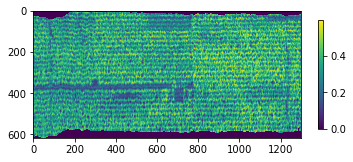`get_wavelength`(target_band, spyfile=None)[source]

Returns actual wavelength of the closest target band.

Parameters
• target_band (`int` or `float`) – the target band to retrieve wavelength number for (required).

• spyfile (`SpyFile` object, optional) – The datacube being accessed and/or manipulated; if `None`, uses `hstools.spyfile` (default: `None`).

Returns

key_wavelength (`float`) – wavelength of the closest target band (`target_band`).

Return type

`float`

Example

Load and initialize `hsio`

```>>> from hs_process import hsio
>>> fname_in = r'F:\nigo0024\Documents\hs_process_demo\Wells_rep2_20180628_16h56m_pika_gige_7-Convert Radiance Cube to Reflectance from Measured Reference Spectrum.bip.hdr'
>>> io = hsio(fname_in)
```

Use `hstools.get_wavelength` to find the wavelength value corresponding to the 151st band

```>>> io.tools.get_wavelength(151, io.spyfile)
702.52
```
`load_spyfile`(spyfile)[source]

Loads a `SpyFile` (Spectral Python object) for data access and/or manipulation by the `hstools` class.

Parameters

spyfile (`SpyFile` object) – The datacube being accessed and/or manipulated.

Example

Load and initialize `hsio`

```>>> from hs_process import hsio
>>> fname_in = r'F:\nigo0024\Documents\hs_process_demo\Wells_rep2_20180628_16h56m_pika_gige_7-Convert Radiance Cube to Reflectance from Measured Reference Spectrum.bip.hdr'
>>> io = hsio(fname_in)
```

Load a new datacube using `hstools.load_spyfile`

```>>> io.tools.load_spyfile(io.spyfile)
>>> io.tools.spyfile
Data Source:   'F:\nigo0024\Documents\hs_process_demo\Wells_rep2_20180628_16h56m_pika_gige_7-Convert Radiance Cube to Reflectance from Measured Reference Spectrum.bip'
# Rows:            617
# Samples:        1300
# Bands:           240
Interleave:        BIP
Quantization:  32 bits
Data format:   float32
```
`mask_array`(array, metadata, thresh=None, percentile=None, side='lower')[source]

Creates a masked numpy array based on a threshold value. If `array` is already a masked array, that mask is maintained and the new mask(s) is/ are added to the original mask.

Parameters
• array (`numpy.ndarray`) – The data array to mask.

• thresh (`float` or `list`) – The value for which to base the threshold; if `thresh` is `list` and `side` is `None`, then all values in `thresh` will be masked; if `thresh` is `list` and `side` is not `None`, then only the first value in the list will be considered for thresholding (default: `None`).

• percentile (`float`) – The percentile of pixels to mask; if `percentile` = 95 and `side` = ‘lower’, the lowest 95% of pixels will be masked prior to calculating the mean spectra across pixels (default: `None`; range: 0-100).

• side (`str`) – The side of the threshold for which to apply the mask. Must be either ‘lower’, ‘upper’, or `None`; if ‘lower’, everything below the threshold will be masked; if `None`, only the values that exactly match the threshol will be masked (default: ‘lower’).

Returns

2-element `tuple` containing

• array_mask (`numpy.ndarray`): The masked `numpy.ndarray` based on the passed threshold and/or percentile value.

• metadata (`dict`): The modified metadata.

Example

Load and initialize `hsio`

```>>> from hs_process import hsio
>>> fname_in = r'F:\nigo0024\Documents\hs_process_demo\Wells_rep2_20180628_16h56m_pika_gige_7-Convert Radiance Cube to Reflectance from Measured Reference Spectrum.bip.hdr'
>>> io = hsio(fname_in)
```

Retrieve the image band at 800 nm using `hstools.get_band` and `hsio.spyfile.open_memmap`

```>>> band = io.tools.get_band(800)
>>> array = io.spyfile.open_memmap()[:, :, band]
```

Create a masked array of all values below the 75th percentile via `hstools.mask_array`

```>>> array_mask, metadata = io.tools.mask_array(array, io.spyfile.metadata, percentile=75, side='lower')
```

See that the “history” tage in the `metadata` has been modified

```>>> metadata['history'][-158:]
"hs_process.mask_array[<label: 'thresh?' value:None; label: 'percentile?' value:75; label: 'side?' value:lower; label: 'unmasked_pct?' value:24.9935170178282>]"
```

Visualize the unmasked array using `hsio.show_img`. Set `vmin` and `vmax` to ensure the same color scale is used in comparing the masked vs. unmasked arrays.

```>>> vmin = array.min()
>>> vmax = array.max()
>>> io.show_img(array, vmin=vmin, vmax=vmax)
```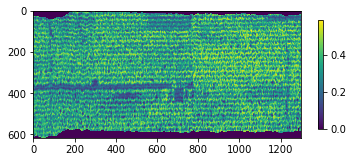Visualize the unmasked array using `hsio.show_img`

```>>> io.show_img(array_mask, vmin=vmin, vmax=vmax)
```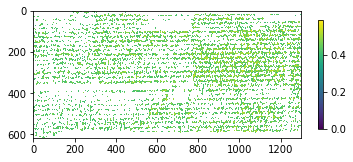`mean_datacube`(spyfile, mask=None)[source]

Calculates the mean spectra for a datcube; if `mask` is passed (as a `numpy.ndarray`), then the mask is applied to `spyfile` prior to computing the mean spectra.

Parameters:
spyfile (`SpyFile` object or `numpy.ndarray`): The

mask (`numpy.ndarray`): the mask to apply to `spyfile`; if

`mask` does not have similar dimensions to `spyfile`, the first band (i.e., first two dimensions) of `mask` will be repeated n times to match the number of bands of `spyfile` (default: `None`).

Returns:

3-element `tuple` containing

• spec_mean (`SpyFile.SpyFile` object): The mean spectra from the input datacube.

• spec_std (`SpyFile.SpyFile` object): The standard deviation of the spectra from the input datacube.

• datacube_masked (`numpy.ndarray`): The masked `numpy.ndarray`; if `mask` is `None`, `datacube_masked` is identical to the `SpyFile` data array.

Example:

Load and initialize `hsio`

```>>> from hs_process import hsio
>>> fname_in = r'F:\nigo0024\Documents\hs_process_demo\Wells_rep2_20180628_16h56m_pika_gige_7-Convert Radiance Cube to Reflectance from Measured Reference Spectrum.bip.hdr'
>>> io = hsio(fname_in)
```

Retrieve the image band at 800 nm using `hstools.get_band` and `hsio.spyfile.open_memmap`, then mask out all pixels whose value falls below the 75th percentile.

```>>> band = io.tools.get_band(800)
>>> array = io.spyfile.open_memmap()[:, :, band]
```

Calculate the spectral mean from the remaining (i.e., unmasked) pixels using `hstools.mean_datacube`.

```>>> spec_mean, spec_std, datacube_masked = io.tools.mean_datacube(io.spyfile, mask=array_mask)
```

Save using `hsio.write_spec` and `hsio.write_cube`, then load into Spectronon software for visualization.

```>>> fname_hdr_spec = r'F:\nigo0024\Documents\hs_process_demo\hstools\Wells_rep2_20180628_16h56m_pika_gige_7-mean_800nm_75th.spec.hdr'
>>> fname_hdr_cube = r'F:\nigo0024\Documents\hs_process_demo\hstools\Wells_rep2_20180628_16h56m_pika_gige_7-mean_800nm_75th.bip.hdr'
Saving F:
```
igo0024Documentshs_process_demohstoolsWells_rep2_20180628_16h56m_pika_gige_7-mean_800nm_75th.spec
```>>> io.write_cube(fname_hdr_cube, datacube_masked, metadata=metadata)
Saving F:
```

igo0024Documentshs_process_demohstoolsWells_rep2_20180628_16h56m_pika_gige_7-mean_800nm_75th.bip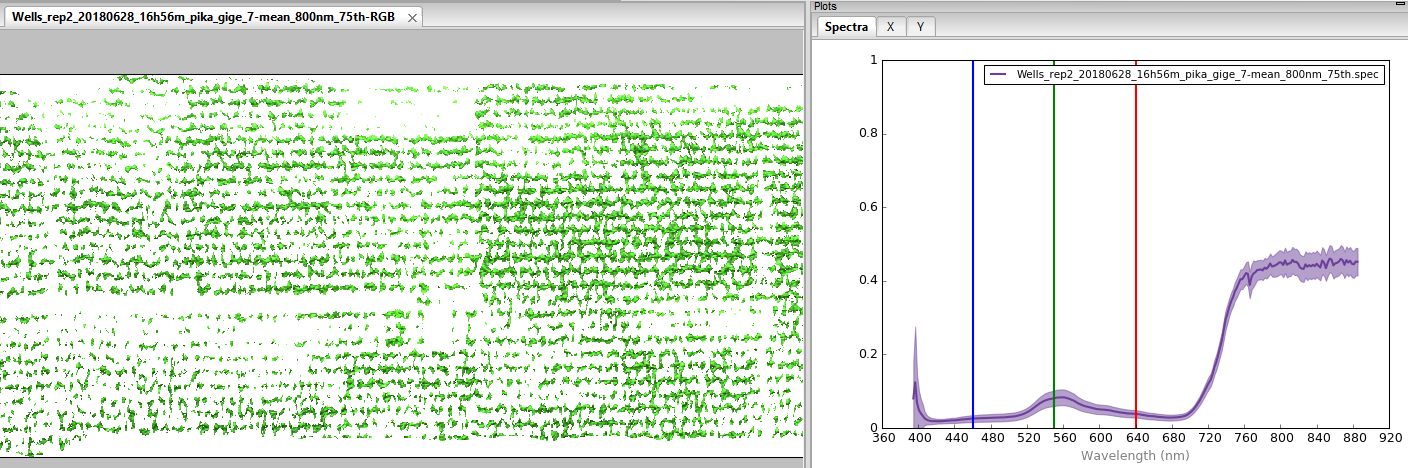`modify_meta_set`(meta_set, idx, value)[source]

Modifies metadata “set” (i.e., string representation of a Python set; common in .hdr files) by converting string to list, then adjusts the value of an item by its index.

Parameters
• meta_set (`str`) – the string representation of the metadata set

• idx (`int`) – index to be modified; if `None`, the whole meta_set is returned (default: `None`).

• value (`float`, `int`, or `str`) – value to replace at idx

Returns

set_str (`str`): Modified metadata set string.

Return type

`str`

Example

Load and initialize `hsio`

```>>> from hs_process import hsio
>>> fname_in = r'F:\nigo0024\Documents\hs_process_demo\Wells_rep2_20180628_16h56m_pika_gige_7-Convert Radiance Cube to Reflectance from Measured Reference Spectrum.bip.hdr'
>>> io = hsio(fname_in)
```

Retrieve the “map info” set from the metadata via `hstools.get_meta_set`

```>>> map_info_set = io.spyfile.metadata['map info']
>>> map_info_set
['UTM',
'1.0',
'1.0',
'441357.287073',
'4855944.7717699995',
'0.04',
'0.04',
'15',
'T',
'WGS-84',
'units  meters',
'rotation  0.000']
```

Modify the value at index position 4 from `4855944.7717699995` to `441300.2` using `hstools.modify_meta_set`.

```>>> io.tools.modify_meta_set(map_info_set, idx=4, value=441300.2)
'{UTM, 1.0, 1.0, 441357.287073, 441300.2, 0.04, 0.04, 15, T, WGS-84, units  meters, rotation  0.000}'
```
`plot_histogram`(array, fname_fig=None, title=None, xlabel=None, percentile=90, bins=50, fontsize=16, color='#444444')[source]

Plots a histogram with the percentile value labeled.

Parameters
• array (`numpy.ndarray`) – The data array used to create the histogram for; if `array` is masked, the masked pixels are excluded from the histogram.

• fname_fig (`str`, optional) – The filename to save the figure to; if `None`, the figure will not be saved (default: `None`).

• title (`str`, optional) – The plot title (default: `None`).

• xlabel (`str`, optional) – The x-axis label of the histogram (default: `None`).

• percentile (`scalar`, optional) – The percentile to label and illustrate on the histogram; if `percentile` = 90, the band/spectral index value at the 90th percentile will be labeled on the plot (default: 90; range: 0-100).

• bins (`int`, optional) – Number of histogram bins (default: 50).

• fontsize (`scalar`) – Font size of the axes labels. The title and text annotations will be scaled relatively (default: 16).

• color (`str`, optional) – Color of the histogram columns (default: “#444444”)

Returns

Figure object showing the histogram.

Return type

fig (`matplotlib.figure`)

Example

Load and initialize `hsio`

```>>> from hs_process import hsio
>>> fname_in = r'F:\nigo0024\Documents\hs_process_demo\Wells_rep2_20180628_16h56m_pika_gige_7-Convert Radiance Cube to Reflectance from Measured Reference Spectrum.bip.hdr'
>>> io = hsio(fname_in)
```

Retrieve the image band at 800 nm using `hstools.get_band` and `hsio.spyfile.open_memmap`

```>>> band = io.tools.get_band(800)
>>> array = io.spyfile.open_memmap()[:, :, band]
```

Create a masked array of all values below the 5th percentile via `hstools.mask_array`

```>>> array_mask, metadata = io.tools.mask_array(array, io.spyfile.metadata, percentile=5, side='lower')
```

Visualize the histogram of the unmasked pixels (i.e., those greater than the 5th percentile) using `hstools.plot_histogram`

```>>> title = 'Reflectance at 800 nm'
>>> xlabel = 'Reflectance (%)'
>>> fig = io.tools.plot_histogram(array_mask, title=title, xlabel=xlabel)
```